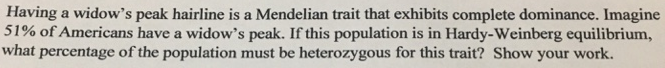# Problem: Having a widow's peak hairline is a Mendelian trait that exhibits complete dominance. Imagine 51% of Americans have a widow's peak. If this population is in Hardy-Weinber equilibrium, what percentage of the population must be heterozygous for this trait?

###### FREE Expert Solution

If this trait is at Hardy-Weinberg equilibrium, the relative population carrying the homozygous dominant genotype, p2 is 0.51. This makes p equal to 0.71.

Remember that p + q = 1. Rearranging the equation, q can be computed as 1 - p. That means,

q = 1 - 0.71 = 0.29.###### Problem Details

Having a widow's peak hairline is a Mendelian trait that exhibits complete dominance. Imagine 51% of Americans have a widow's peak. If this population is in Hardy-Weinber equilibrium, what percentage of the population must be heterozygous for this trait?